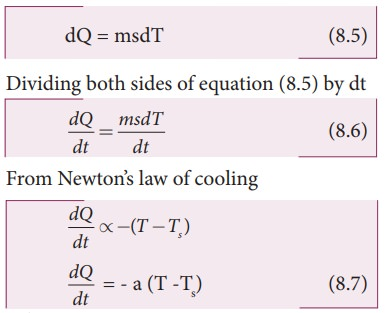Home | | Physics 11th std | NewtonŌĆÖs law of cooling

# NewtonŌĆÖs law of cooling

NewtonŌĆÖs law of cooling states that the rate of loss of heat of a body is directly proportional to the di’¼Ćerence in the temperature between that body and its surroundings .

NewtonŌĆÖs law of cooling

NewtonŌĆÖs law of cooling states that the rate of loss of heat of a body is directly proportional to the di’¼Ćerence in the temperature between that body and its surroundings .The negative sign indicates that the quantity of heat lost by liquid goes on decreasing with time. Where,

T = Temperature of the object

Ts = Temperature of the surroundingFrom the graph in Figure 8.12 it is clear that the rate of cooling is high initially and decreases with falling temperature.

Let us consider an object of mass m and specific heat capacity s at temperature T. Let Ts be the temperature of the surroundings. If the temperature falls by a small amount dT in time dt, then the amount of heat lost is,Where a is some positive constant.

From equation (8.6) and (8.7)Where b1 is the constant of integration. taking exponential both sides, we gethere b2 = eb1 = constant

### EXAMPLE 8.8

A hot water cools from 92┬░C to 84┬░C in 3 minutes when the room temperature is 27┬░C. How long will it take for it to cool from 65┬░C to 60┬░C?

The hot water cools 8┬░C in 3 minutes. The average temperature of 92┬░C and 84┬░C is 88┬░C. This average temperature is 61┬░C above room temperature. Using equation (8.8)Similarly the average temperature of 65┬░C and 60┬░C is 62.5┬░C. The average temperature is 35.5┬░C above the room temperature. Then we can writeBy diving both the equation, we getStudy Material, Lecturing Notes, Assignment, Reference, Wiki description explanation, brief detail
11th Physics : UNIT 8 : Heat and Thermodynamics : NewtonŌĆÖs law of cooling |